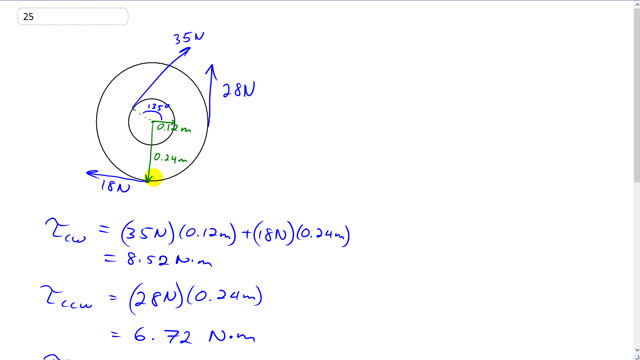## You are here

Question:

Calculate the net torque about the axle of the wheel shown in Fig. 8–42. Assume that a friction torque of $0.60 \textrm{ m}\cdot\textrm{N}$ opposes the motion.Figure 8-42.

Giancoli, Douglas C., Physics: Principles with Applications, 7th Ed., ©2014. Reprinted by permission of Pearson Education Inc., New York.
The question will be visible after logging in, as required by Pearson Education Inc.

$-1.2 \textrm{ N m}$Hi barnettlenworth, thank you for the comment. You're quite right that Netwtons times meters makes units of energy - Joules. However, it's nevertheless typical to write units of torque as $\textrm{N}\cdot \textrm{m}$, and it's understood that since $\textrm{J}$ isn't used instead, that $\textrm{N}\cdot \textrm{m}$ represents torque.